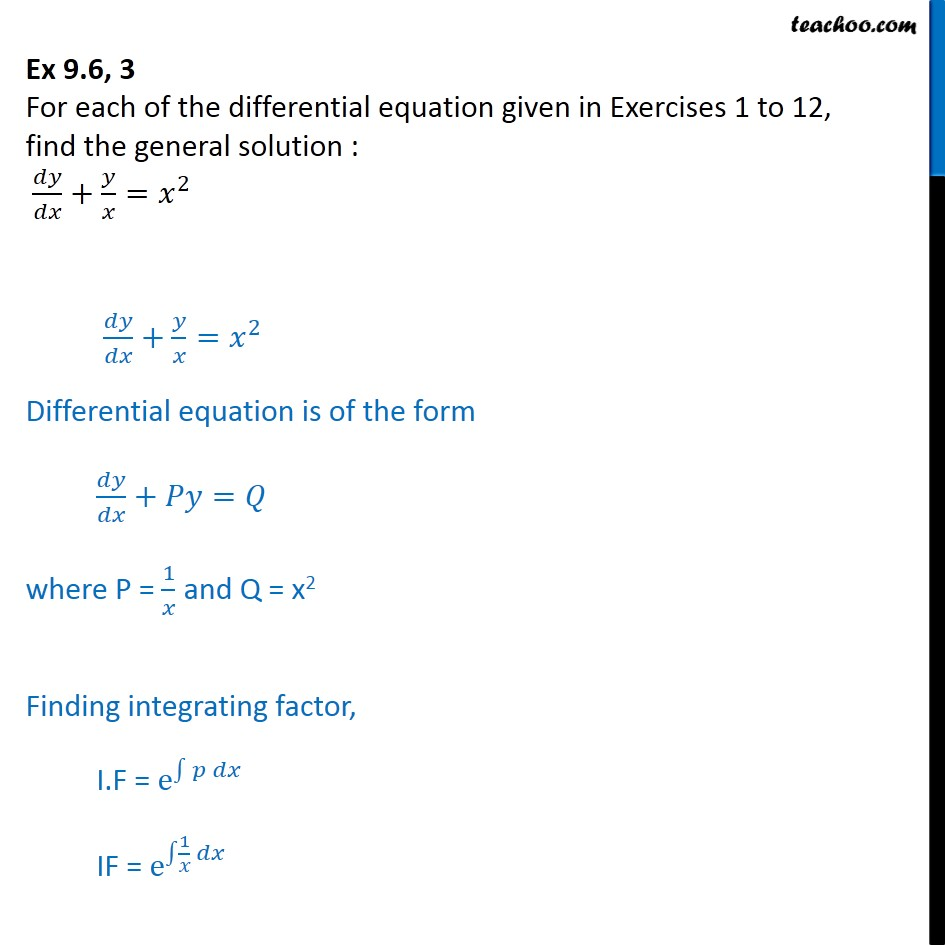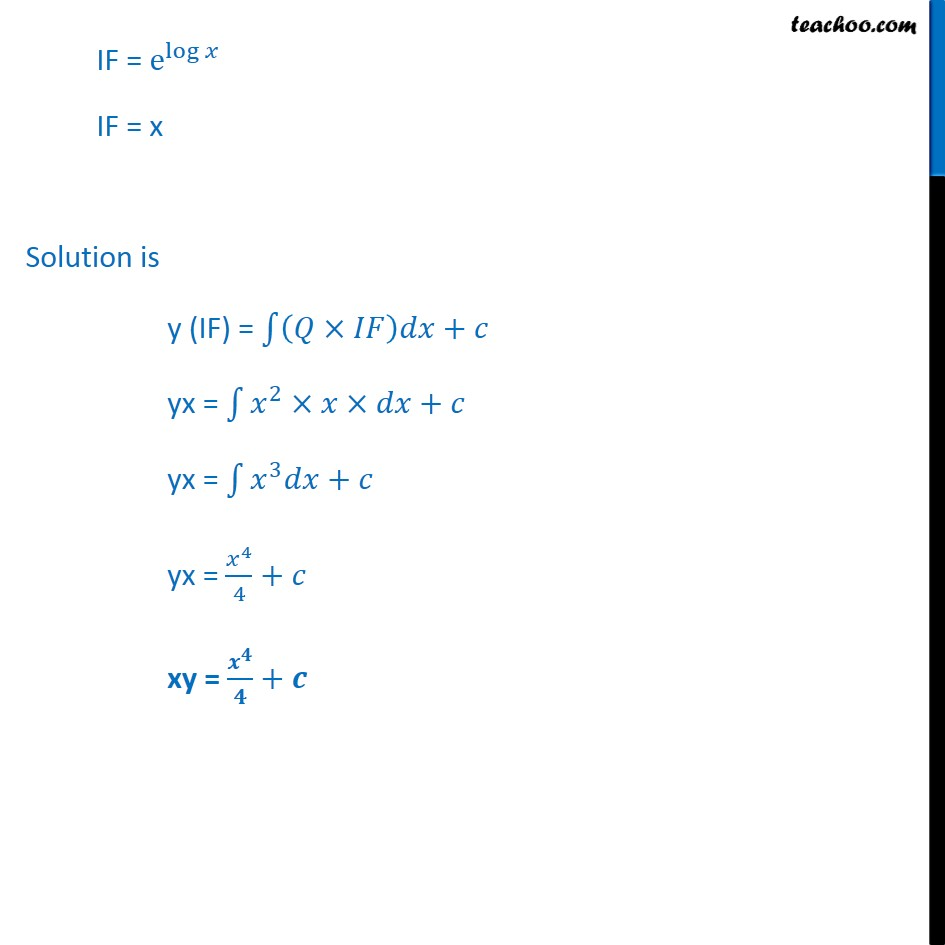Ex 9.5

Chapter 9 Class 12 Differential Equations
Serial order wiseLearn in your speed, with individual attention - Teachoo Maths 1-on-1 Class

### Transcript

Ex 9.5, 3 For each of the differential equation given in Exercises 1 to 12, find the general solution : + = 2 + = 2 Differential equation is of the form + = where P = 1 and Q = x2 Finding integrating factor, I.F = e IF = e 1 IF = e log IF = x Solution is y (IF) = + yx = 2 + yx = 3 + yx = 4 4 + xy = +